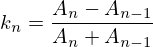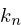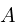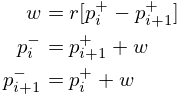Tract

# Tract

## Overview

Tract implements a rudimentary physical model of vocal tract for use in articulatory synthesis. It is based on the classic kelly-lochbaum model developed at Bell Labs in the early 60s. In recent years, such a model has gotten new breath (so to speak) in programs like Pink Trombone, from which this particular was derived from.

In order to be both "physically based" and practically computable, the model crudely pretends that our vocal tract is a series of cylindrical tubes with varying diameters. Changing the diameters in various ways will result in different phonemes to come out the other side. Almost by magic.

What combinations of diameters produce what kind of phonemes? It's not a straightforward answer, and new methods for finding ideal shapes are still an ongoing area of research. In the early days, measurements were originally obtained from real vocal tracts!

The tract is a filter that expects some kind of external input. The closer that input resembles the kind of sound our glottis makes, the more vocal-like the output sounds will turn out. In programs like Pink Trombone, an analytical model of the glottis was used as the source signal for it's vocal tract filter.

Compared to Pink Trombone, this model is greatly simplified. Both implement the digital waveguide as a ladder filter consisting of 44 Kelly-Lochbaum scattering junctions. In this model, the nasal component (known as velum control) has been removed. Also removed is the transient generator, used whenever there were blocking interferences in the vocal tract. These can still be found in Voc.

## Tangled files

Tract tangles into two main files: tract.c and tract.h. Defining SK_TRACT_PRIV will expose the struct.

<<tract.c>>=
#include <stdlib.h>
#include <math.h>
#include <string.h>

#ifndef M_PI
#define M_PI 3.14159265358979323846
#endif

#define SK_TRACT_PRIV
#include "tract.h"

<<static_funcdefs>>
<<funcs>>

<<tract.h>>=
#ifndef SK_TRACT_H
#define SK_TRACT_H
#ifndef SKFLT
#define SKFLT float
#endif
<<typedefs>>
<<funcdefs>>
#ifdef SK_TRACT_PRIV
<<structs>>
#endif
#endif

## Struct and Initialization

The core struct is defined as sk_tract.

<<typedefs>>=
typedef struct sk_tract sk_tract;

<<structs>>=
struct sk_tract {
<<sk_tract>>
};

The struct is initialized with sk_tract_init.

<<funcdefs>>=
void sk_tract_init(sk_tract *tr);

<<funcs>>=
void sk_tract_init(sk_tract *tr)
{
<<init>>
calculate_reflections(tr);
}

## Components and Variables

### Glottal and Lip Reflection constants

Glottal and lip reflection constants. These are set to be 0.75 and -0.85, respectively.

<<sk_tract>>=
SKFLT glottal_reflection;
SKFLT lip_reflection;

<<init>>=
tr->glottal_reflection = 0.75;
tr->lip_reflection = -0.85;

### Tract Size

The active size of the vocal tract is determined by a variable n. It can have a maximum value of 44.

<<sk_tract>>=
int n;

<<init>>=
tr->n = 44;

### Diameters

The diameters array are inputs available to manipulate the vocal tract.

<<sk_tract>>=
SKFLT diameter;

<<init>>=
memset(tr->diameter, 0, 44 * sizeof(SKFLT));
<<initial_shape>>

The diameters get set to an initial shape. This is the one Neil Thapen uses in Pink Trombone:

<<initial_shape>>=
{
int i;

for(i = 0; i < tr->n; i++) {
SKFLT diameter = 0;
if(i < 7 - 0.5) {
diameter = 0.6;
} else if( i < 12) {
diameter = 1.1;
} else {
diameter = 1.5;
}

tr->diameter[i] = diameter;
}
}

### Areas

Diameters get converted into areas and stored in the array A, and areas get converted into reflection coefficients stored in the array reflection.

<<sk_tract>>=
SKFLT A;
SKFLT reflection;

<<init>>=
memset(tr->A, 0, 44 * sizeof(SKFLT));
memset(tr->reflection, 0, 44 * sizeof(SKFLT));

### Scattering Junction Outputs and Bidirectional Delay Line

Each scattering junction has an left and right output corresponding to the direction of pressure, as well as filter memory. The junction output arrays are known as junction_outL and junction_outR, respectively. Additional Left/Right arrays are used to implement the bidirectional delay line for the underlying waveguide.

<<sk_tract>>=
SKFLT junction_outL;
SKFLT L;
SKFLT junction_outR;
SKFLT R;

<<init>>=
memset(tr->junction_outL, 0, 44 * sizeof(SKFLT));
memset(tr->L, 0, 44 * sizeof(SKFLT));
memset(tr->junction_outR, 0, 44 * sizeof(SKFLT));
memset(tr->R, 0, 44 * sizeof(SKFLT));

### User-Supplied Shape Callback

A user-supplied shape callback can be used to shape the diameters at audio-rate, allowing for things like smoothing filters. A user-data void struct is also included here.

<<sk_tract>>=
void *ud;
void (*shape)(sk_tract *, SKFLT *, void *);

<<init>>=
tr->shape = NULL;
tr->ud = NULL;

### Use Diameters Flag

The use_diameters flag can be used to enable/disable the diameter control. If disabled, areas can be directly manipulated.

<<sk_tract>>=
int use_diameters;

<<init>>=
sk_tract_use_diameters(tr, 1);

It is set with the function sk_tract_use_diameters, where mode is true (1) or false (0).

<<funcdefs>>=
void sk_tract_use_diameters(sk_tract *tr, int mode);

<<funcs>>=
void sk_tract_use_diameters(sk_tract *tr, int mode)
{
tr->use_diameters = mode;
}

## Diameter Manipulation

The vocal tract is controlled by mainpulating the underlying diameter sizes. These can be directly accessed via sk_tract_diameters.

<<funcdefs>>=
SKFLT* sk_tract_diameters(sk_tract *tr);

<<funcs>>=
SKFLT* sk_tract_diameters(sk_tract *tr)
{
return tr->diameter;
}

The number of diameters can be retrieved with sk_tract_size. Usually, this is 44.

<<funcdefs>>=
int sk_tract_size(sk_tract *tr);

<<funcs>>=
int sk_tract_size(sk_tract *tr)
{
return tr->n;
}

More often than not, one wants to apply sample-accurate smoothing to the diameters rather than work them directly. This is done using a callback interface, known as a shaper.

The function sk_tract_shaper sets up a shaper callback. It takes in the shaper callback as well as any external user data needed to manage state in that callback.

This function gets called at every sample, and takes in three arguments: the sk_tract struct, the output array to write to, and the externally managed user data.

In practice, one potential approach is to use a filterbank of 44 smoothing filters to control the diameter shapes. This allows diameters to be controlled without producing any artificats caused by large discontinuities.

<<funcdefs>>=
void sk_tract_shaper(sk_tract *tract,
void (*shape)(sk_tract *, SKFLT *, void *),
void *ud);

<<funcs>>=
void sk_tract_shaper(sk_tract *tract,
void (*shape)(sk_tract *, SKFLT *, void *),
void *ud)
{
tract->shape = shape;
tract->ud = ud;
}

Neil Thapen's Pink Trombone employs a curious "tongue control" functionality, which allows one use 2 dimensions of control to shape the entire tract. This is also the underlying control mechanism for Voc.

This behavior is ported in the function sk_tract_tongue_shape, where position and diameter are both normalized floating point values.

<<funcdefs>>=
void sk_tract_tongue_shape(sk_tract *tract,
SKFLT position,
SKFLT diameter);

<<funcs>>=
static void set_diameters(sk_tract *tract,
int lip_start,
int tip_start,
SKFLT tongue_index,
SKFLT tongue_diameter,
SKFLT *diameters)
{
int i;
SKFLT t;
SKFLT fixed_tongue_diameter;
SKFLT curve;
int grid_offset = 0;

for(i = blade_start; i < lip_start; i++) {
t = 1.1 * M_PI *
fixed_tongue_diameter = 2+(tongue_diameter-2)/1.5;
curve = (1.5 - fixed_tongue_diameter + grid_offset) * cos(t);
if(i == blade_start - 2 || i == lip_start - 1) curve *= 0.8;
if(i == blade_start || i == lip_start - 2) curve *= 0.94;
diameters[i] = 1.5 - curve;
}
}

void sk_tract_tongue_shape(sk_tract *tract,
SKFLT position,
SKFLT diameter)
{
position = 12 + 16.0 * position;
diameter = 3.5 * diameter;
set_diameters(tract, 10, 39, 32,
position, diameter, tract->diameter);
}

## Area Manipulation

Sometimes it might be more adventageous to set the areas directly, rather than using the diameters (which are then squared and set as the area).

To use areas directly, diameter control must be turned off. This done by setting the use_diameters flag to be false via sk_tract_use_diameters.

Area shapes can be set using the function sk_tract_set_area_shape. This will set the shape of the area to be an array of size sz. If the array is larger than the current tract size, it will be truncated. If it is smaller, the last values will be padded with the last tract sample in the array.

<<funcdefs>>=
void sk_tract_set_area_shape(sk_tract *tr, SKFLT *areas, int sz);

<<funcs>>=
void sk_tract_set_area_shape(sk_tract *tr, SKFLT *areas, int sz)
{
int n;
SKFLT last;

last = 0;

for (n = 0; n < tr->n; n++) {
if (n >= sz) {
tr->A[n] = last;
} else {
tr->A[n] = areas[n];
last = areas[n];
}
}
}

## Computing Audio

### Tick Function

A single sample of audio is computed with sk_tract_tick. It expects an input signal in, and returns a single sample.

<<funcdefs>>=
SKFLT sk_tract_tick(sk_tract *tract, SKFLT in);

There are two main things that happen here. First, the reflection coefficients for the ladder filter are calculated. Then, the input is computed. The output is then scaled and returned.

Note that tract_compute is called twice, an artifact from Pink Trombone. Most likely this is done to simulate forward and backward propogation.

<<funcs>>=
SKFLT sk_tract_tick(sk_tract *tr, SKFLT in)
{
SKFLT tmp;
SKFLT out;

out = 0;

calculate_reflections(tr);
tmp = 0;

/* compute twice for forwards/backwards propogation */
tmp += tract_compute(tr, in);
tmp += tract_compute(tr, in);

out = tmp * 0.125;

return out;
}

### Calculate Reflections

<<static_funcdefs>>=
static void calculate_reflections(sk_tract *tr);

<<funcs>>=
static void calculate_reflections(sk_tract *tr)
{
int i;
SKFLT *diam;

diam = tr->diameter;

<<shapeit>>
<<calculate_areas>>
<<calculate_reflection_coefficients>>
}

If the shape function exists, call it. If use_diameters is enabled (by default it is), it will pass in the diameters as an output. Otherwise, it will pass in the areas A directly.

<<shapeit>>=
if (tr->shape != NULL) {
if (tr->use_diameters)
tr->shape(tr, tr->diameter, tr->ud);
else
tr->shape(tr, tr->A, tr->ud);
}

The cross-sectional areas are calculated by squaring the input diameters. This will only happen if use_diameters is enabled.

<<calculate_areas>>=
if (tr->use_diameters) {
for(i = 0; i < tr->n; i++) {
tr->A[i] = diam[i] * diam[i];
}
}

The reflection coefficients are calculated from the computed areas. This is the difference between neighboring areas over their sum:Whereis known as the scattering coefficient or reflection coefficient, andare the areas.

(Adapted from Perry Cook's Real Sound Synthesis for interactive Applications, found on pg. 230)

To prevent numerical issues, reflections are sent to a close-to-1 value if the area is exactly 0.

<<calculate_reflection_coefficients>>=
for(i = 1; i < tr->n; i++) {
if(tr->A[i] == 0) {
tr->reflection[i] = 0.999; /* to prevent bad behavior if 0 */
} else {
tr->reflection[i] =
(tr->A[i - 1] - tr->A[i]) / (tr->A[i - 1] + tr->A[i]);
}
}

### Tract Compute

A single pass of tract computation is done with tract_compute.

<<static_funcdefs>>=
static SKFLT tract_compute(sk_tract *tr, SKFLT in);

<<funcs>>=
static SKFLT tract_compute(sk_tract *tr, SKFLT in)
{
SKFLT  r, w;
int i;
SKFLT out;

out = 0;

<<initial_junction_outputs>>
<<compute_scattering_junctions>>
<<update_delay_lines>>
<<get_output>>

return out;
}

The tract has air flow moving in two directions. The right direction is glottis. The left direction are the lips.

<<initial_junction_outputs>>=
tr->junction_outR = tr->L * tr->glottal_reflection + in;
tr->junction_outL[tr->n - 1] = tr->R[tr->n - 1] * tr->lip_reflection;

First, the left/right junction outputs are computed.

The variable names used here are adapted from Jack Mullen's PhD dissertation, on the section on BiDirectional Waveguide Composition, in section 2.5.2, figure 2.77:<<compute_scattering_junctions>>=
for(i = 1; i < tr->n; i++) {
r = tr->reflection[i];
w = r * (tr->R[i - 1] + tr->L[i]);
tr->junction_outR[i] = tr->R[i - 1] - w;
tr->junction_outL[i - 1] = tr->L[i] + w;
}

The left and right delay lines are updated as attenuated versions of the junction ouputs.

<<update_delay_lines>>=
for(i = 0; i < tr->n; i++) {
tr->R[i] = tr->junction_outR[i]*0.999;
tr->L[i] = tr->junction_outL[i]*0.999;
}

The output signal is the last sample in the right-moving delay line.

<<get_output>>=
out = tr->R[tr->n - 1];